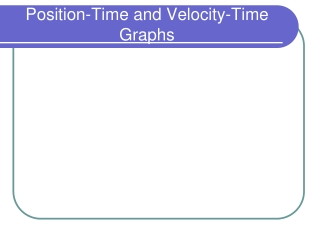DownloadDownload PresentationPosition-Time and Velocity-Time Graphs

# Position-Time and Velocity-Time Graphs

Télécharger la présentation## Position-Time and Velocity-Time Graphs

- - - - - - - - - - - - - - - - - - - - - - - - - - - E N D - - - - - - - - - - - - - - - - - - - - - - - - - - -
##### Presentation Transcript

1. Position-Time and Velocity-Time Graphs

2. Questions for Consideration • What is a position-time graph? • What is a velocity-time graph? • How do features on one graph translate into features on the other?

3. Position-Time Graphs • Show an object’s position as a function of time. • x-axis: time • y-axis: position

4. Position-Time Graphs • Imagine a ball rolling along a table, illuminated by a strobe light every second. • You can plot the ball’s position as a function of time. 0 s 1 s 2 s 3 s 4 s 5 s 6 s 7 s 8 s 9 s 10 s

5. 10 9 8 7 6 5 4 3 2 1 1 2 3 4 5 6 7 9 10 8 Position-Time Graphs position (cm) time (s)

6. 10 9 8 7 6 5 position (cm) 4 3 2 1 time (s) 1 2 3 4 5 6 7 9 10 8 Position-Time Graphs • What are the characteristics of this graph? • Straight line, upward slope • What kind of motion created this graph? • Constant speed

7. Position-Time Graphs • Each type of motion has a characteristic shape on a P-T graph. • Constant speed • Zero speed (at rest)

8. time (s) time (s) pos. (m) pos. (m) Constant speed in positive direction. Constant speed in negative direction. Position-Time Graphs • Constant speed is represented by a straight segment on the P-T graph.

9. time (s) pos. (m) A horizontal segment means the object is at rest. Position-Time Graphs • No movement (object is stopped) is represented by a straight segment on the P-T graph.

10. 50 change in y slope = 40 change in x 30 position (m) (30 m – 10 m) 20 slope = (30 s – 0 s) 10 (20 m) 10 20 30 40 slope = (30 s) time (s) Position-Time Graphs • The slope of a P-T graph is equal to the object’s velocity in that segment. slope = 0.67 m/s

11. Velocity-Time Graphs • A velocity-time (V-T) graph shows an object’s velocity as a function of time. • A horizontal line = constant velocity.

12. + velocity (m/s) time (s) - Velocity-Time Graphs • A horizontal line on the V-T graph means constant velocity. Object is moving at a constant velocity in the + direction

13. + velocity (m/s) time (s) - Velocity-Time Graphs • A horizontal line on the V-T graph means constant velocity. Object is moving at a constant velocity in - direction

14. + velocity (m/s) time (s) - Velocity-Time Graphs • If an object isn’t moving, its velocity is zero. Object is at rest